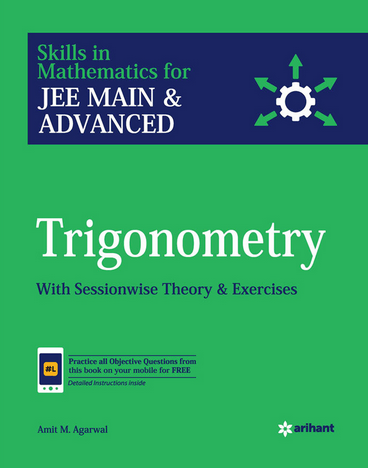Trigonometry (Weightage 4%)   Share

Topics from Trigonometry

• Trigonometrical identities and equations (67 concepts)
• Trigonometrical functions, Inverse trigonometrical functions and their properties (30 concepts)
• Height and distance (4 concepts)

Important Books for Trigonometry

•••Exams
Articles
Questions